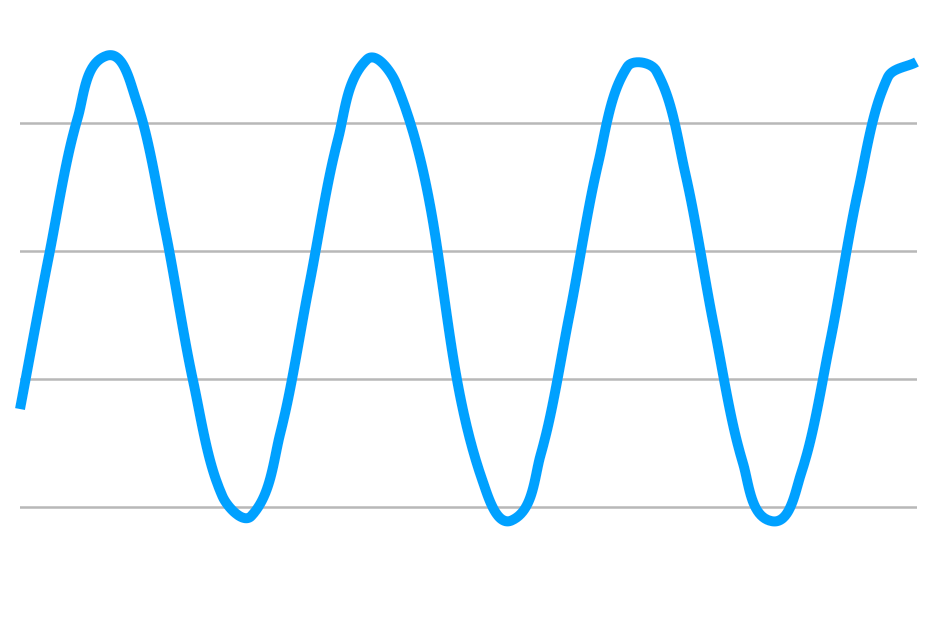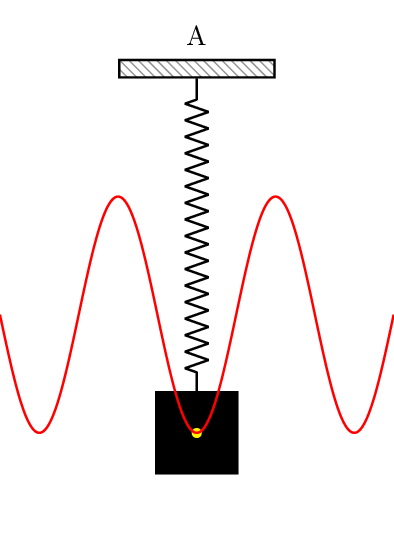Search
•Edward Sanchez

# Spring Animations

I was working on some prototypes which required me to animate properties which were not supported by UIKit animators. So I was left with no choice but to build my own animator.

No worthy animator exists without springs. And I knew nothing about springs beyond "they are bouncy!". I had a lot to learn.

I remember looking at the properties of a spring (mass, stiffness, damping, velocity) and had very little idea of what they meant. Creating a spring was more of a guessing game inputting numbers randomly until I got something that worked.

Well let me tell you, it took a LOT of googling to understand these damn, I mean awesome, things! Most articles were super advanced and assumed a greater understanding of math than I currently have. So I wanted to try to write my own post, targeted at designers and developers who, like me, might not have prior understanding of these principles.

I’m going to try to get as basic as possible, which at times may be too basic for some of you.

I’m also going to explain why stiffness and mass don’t need to be manually set, but it helps to understand springs in order to understand why that is.

# Hooke’s Law / Harmonic Oscillators

First of all, let’s forget about the spring in the real world. The springs we know have a size, a thickness and often have something attached at the end of them.

But in mathematical terms I spring is what’s called a Harmonic Oscillator. Basically an infinite wavy curve like this:To “draw” this curve with math you obviously need to plot the position over time.

So let’s break that down.

If we're drawing a wavy line, it means there's a change in velocity going on - acceleration and deceleration. And if there’s acceleration then there’s gotta be a force making it go faster and slower.

A resting spring has no force, so you need to “activate” it by pulling it. How far you pull is called our displacement.

Now that it’s pulled, there’s a force pulling it back to its original position. And there’s a special formula to get the force of a spring based on Hooke’s Law.

F = -kx

k is a stiffness coefficient

x is the displacement value (how far the object moved from previous value)

Force = Negative Stiffness Value * Displacement value

Notice that mass is not a part of this force. All we need to define a spring’s force is how stiff it is.

Now we have the force, so we can calculate the acceleration.

The formula for acceleration is:

a = F ÷ m

Acceleration = Force ÷ Mass

The mass will be a constant, say 1, since we’re assuming our object is not changing weight.

We know our Force is -kx, so if we replace F we get:

a = (-kx) ÷ m

Acceleration = (Negative Stiffness × Displacement) ÷ Mass

Now we know the acceleration, we can calculate the velocity of our object at any given time.

To accelerate velocity you need to add the acceleration rate to the previous velocity.

v1 = v0 + a × t

New Velocity = Previous Velocity + (Acceleration × Time Interval)

The time interval when it comes to computers is normally set in frames per second (hertz).

60 frames per second = 1÷ 60 = 0.016666 seconds

Finally, you can calculate the new position.

p1 = p0 + v × t

New Position = Old Position + New Velocity × Time Interval

Do you see how that never-ending wave is formed? The displacement (x) affects our force since F = -kx. So that means that the force will change based on how far it’s displaced, and if the force changes the acceleration changes too, and if the acceleration changes the velocity changes and the displacement changes, which affects the force…  🤯

All it means is that this will go on forever making the oscillator curve with nothing to stop it. Which is obviously not what we want in an animation.And that’s where damping comes in...

## Damping Coefficient

In nature, no spring is perfect like that. There are forces at play like friction and heat. So the springs we are familiar with always stop at some point. That stopping force is called damping.

The damping force formula is:

F = -dv

Force = Negative Damping Coefficient × Velocity

## Damping Ratio

The necessary force to damp a spring varies on its stiffness and its mass. So, a much easier way to deal with it is to calculate a damping ratio (0 to 1). The greater the fraction, the less bouncy it is. This is the formula to calculate the damping ratio.

ζ = 2 × m × (√k÷m) × d

Damping Ratio = 2 × Mass × (√Stiffness÷Mass) × Damping Coefficient

Damping Ratios also get some fancy names depending on their value:

• Critically damped Damping Ratio = 1 (No bounce at all - The most common in Apple UI)

• Over Damped Damping Ratio > 1  (Under bounce, very stiff, Not a good look, not often used in UI animation)

• Under Damped Damping Ratio < 1  (The smaller the number the bouncier it gets and the more likes you get on Dribbble.)

Back to our formula, now you have the dampening force, you just add it to your spring force, and your spring will stop.

With the new damped force, our acceleration formula looks like this.

a = (-kx) + (-dv) ÷ m

Acceleration = (Negative Stiffness × Displacement) + (Negative Damping Coefficient × Velocity) ÷ Mass

And so you get a spring that looks more like this:## Time

Notice that time is not a variable that we’re setting, and normally when you’re animating something you want to tell it how long it’s going to animate for.

Well, I’m sorry to tell you that springs don’t really care about time, which for springs is referred to as "Relaxation Time". They’ll just take however long it takes them to come to a stop. And that’s a problem, because we think of UI animations in terms of how long they'll last.

Luckily, math is on our side. Your mass, stiffness, and damping all can influence the time it’ll take for the spring to "rest".

The formula for relaxation time took me a long long time to find. Wikipedia and most of the internet has a formula that does not at all work for me in practice.

It's almost impossible to get an exact relaxation time because the spring can continue moving minutely infinitely, the same way you can divide a number by 2 for ever and ever.

So what you're looking for then is when will the spring reach a fraction of the initial amplitude, in other words, when does it look like it stopped moving.

Let's say the fraction of the initial amplitude we want is 1/1500. Here's the formula to find how long it will take for the spring to reach that amplitude:

t = (2 × m × ln(1500)) ÷ d

Time = 2 × Mass × NaturalLog(1500) × Damping Coefficient

That means you can technically control the mass / stiffness / damping to match the approximate time you want the spring to come to a rest.

### Setting a Spring Using Duration and Damping Ratio

Like I said in the beginning, you don't really need to worry about mass and stiffness on an animation. It's unintuitive and mostly what you care about is how long the animation will last and how springy the animation will be.

But how can you calculate the mass, stiffness and damping coefficient given a duration and damping ratio?

To make things simple, you can set one of the values as a constant. I’ll pick mass, and set it to 1 (the number here does not at all change the final spring since damping and stiffness are recalculated to overcome the mass).

That leaves us with two values to calculate; our stiffness and damping coefficient.

I want our damping ratio to be 0.9 (remember this is the ratio as explained above, not the coefficient, the closer to 1 the less bouncy it is), and our animation to last 1 second.

Stiffness

k = (m  × ln(1500)²) / t²  × ζ²

stiffness = (Mass × NaturalLog(1500)²) × Time²  × Damping Ratio²

stiffness = 43.32

Damping Coefficient

c = 2 × ζ × (√k÷m) × m

Damping Coefficient = 2 × Damping Ratio × (√stiffness ÷ mass) × m

dampingCoefficient = 11.84

So, if you set your spring with a damping coefficient of 11.84, mass of 1, stiffness 43.32, it will rest in approximately 1 second and have a damping ratio of 0.9.

### Swift

I’ve been doing all this in swift and made a little git gist with the formulas.

Note that this is not the actual code or formula used by Apple for UIViewPropertyAnimator(duration: TimeInterval, dampingRatio: CGFloat) method. So if you apply the formulas above and make an animator and play alongside an UIViewPropertyAnimation you’ll see slightly different results.